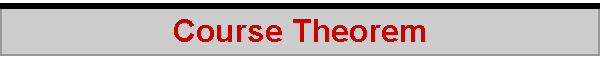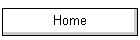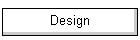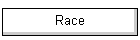About the most important question when racing is, "Can I point better?"  So, what exactly is the theory behind pointing?  Turns out it is both surprisingly simple and surprisingly profound.  Its called the "Course Theorem".  (You can read about it in the books by Ross Garrett, C.A. Marchaj, etc.) We begin by reminding ourselves what happens when sailing upwind -- wind and water...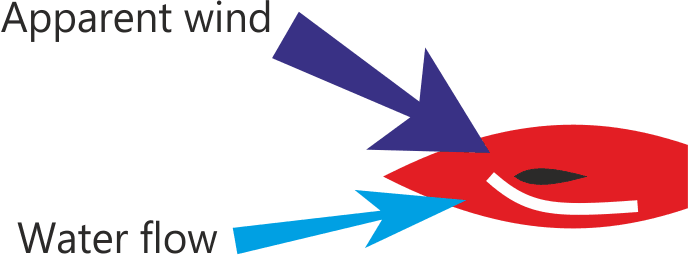Figure 1: Wind and water   The wind on the sails generates lift and drag, and on the boat generates more drag.  Remember that the lift is generated at 90 degrees to the wind, while the drag is in the direction of the wind.  The result is the, ah, "resulting" force, as illustrated.Figure 2: Aero vectors   The boat makes leeway as it moves through the water.  This leeway is the cause of the hydrodynamic lift as contributed by the hull, fin, rudder, and bulb, and naturally there is hydrodynamic drag as well.  Remember that the hydrodynamic lift is generated at 90 degrees to the flow of the water, while the drag is in the direction of the water flow.  And these two forces give us the resulting hydrodynamic force.Figure 3: Hydro vectors   Now this is where things get interesting.  If the boat is in equilibrium, then it is necessarily the case that the aerodynamic force is exactly balanced by the hydrodynamic force.  So, whatever the aerodynamic force is, the hydrodynamic force is opposite to it, and the same magnitude.  This is what Ross Garrett calls the symmetry of sailing.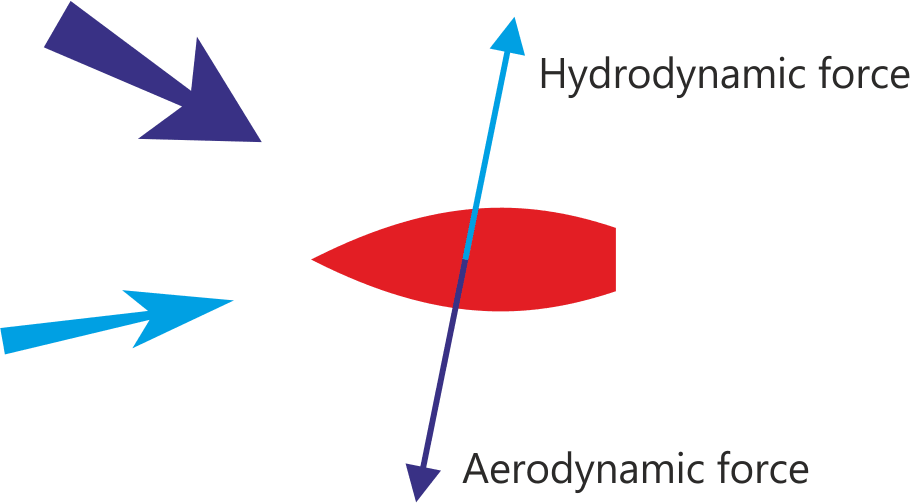Figure 4: The exact balance and symmetry between aero and hydro   Let's check what we mean by "pointing".  It is the angle made between the apparent wind and the boat's motion through the water, its course.  Remember that the boat's heading is not the same as its motion through the water; this is because the boat makes leeway, and though it might be seeming to be heading towards a certain point on the horizon, its course is that it is actually drifting off that point due to leeway.  The sailing angle is the boat's pointing angle, called beta b (leeway is called lambda l).  Now it gets interesting again, because it turns out that beta is the same as the angle between the hydrodynamic drag vector and the aerodynamic drag vector.  This must be -- these two drag vectors are in the direction of their respective causes, the wind and the flow of water, and beta, our pointing angle, is the angle between the apparent wind and the flow of water.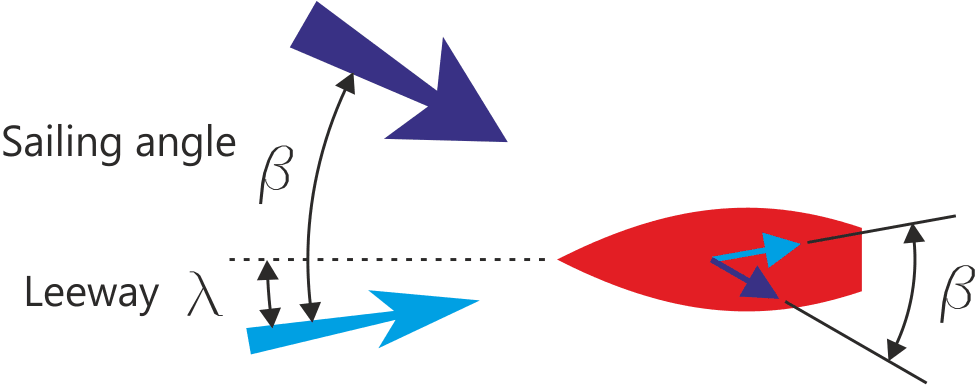Figure 5: Beta, the sailing or pointing angle   So, exactly what is the size of beta?  We start by defining the "drag angle", which is the angle to the resulting force caused by the drag vector acting on the lift vector.  We show the hydrodynamic drag angle, eta e(H), and the aerodynamic drag angle, eta e(A), as the angle given by the ratio of drag to lift.  (Technically, this angle is given by the arc-tan of the ratio.  Airplane folks know it as the gliding angle.  And the drag to lift ratio is the inverse of the the more common lift-to-drag ratio.)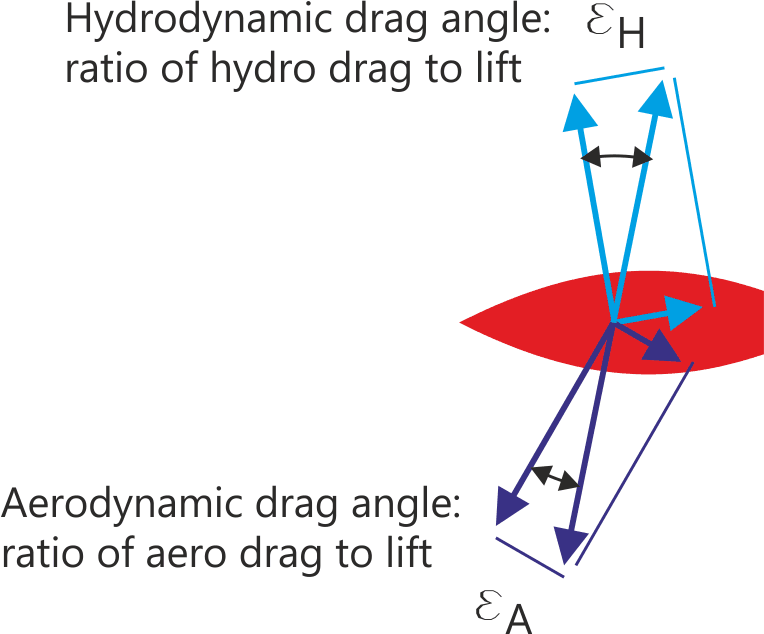Figure 6: Drag angles   And we are there -- the pointing angle or sailing angle, beta b, is equal to the sum of these two drag angles e(H) and e(A).  This is the "course theorem".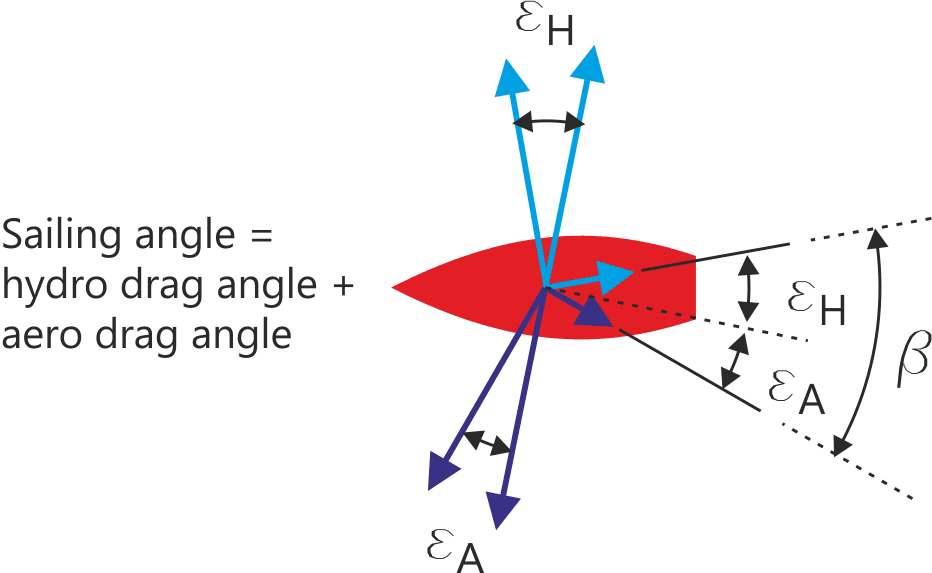Figure 7: Sailing angle is the sum of the two drag angles   You could probably see that coming right from Figure 5, where you saw that the sailing angle was the same as the angle between the two drag vectors. So what?  Well, if you want to point better, you have to reduce the hydrodynamic drag angle, or reduce the aerodynamic drag angle, or both.  You need to immediately appreciate that we are NOT necessarily talking about just reducing drag as such.  Remember, a drag angle is the ratio of drag to lift.  Ah...  To reduce a drag angle, which is in fact a measure of efficiency, you have to increase the efficiency of its lifting component -- which means either increasing lift relative to drag, or reducing drag relative to lift.  Of course, if you can eliminate some drag while keeping lift, well, there you go, you have increased efficiency, lowered the drag angle, and as a result pointed higher! 2012-08-20